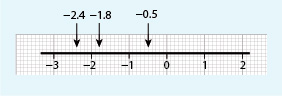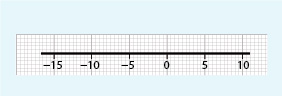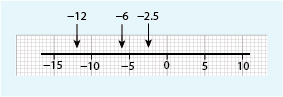Succeed with maths – Part 1

Start this free course now. Just create an account and sign in. Enrol and complete the course for a free statement of participation or digital badge if available.

Free course

# 2 Practical uses of negative numbers

Negative numbers are used in many other practical applications. Banks and other companies, for example, use negative numbers to represent overdrawn accounts or a debt: if you had £50 in your account but then withdrew £60, your account would be overdrawn by £10. This might be recorded as ‘–£10’ or ‘(£10)’. (Brackets are sometimes used to show a negative balance.)

You may also have seen negative numbers on maps, where they are used to record the depth below sea level. If you’re an avid golfer, or enjoy following it on television, you’ve probably noticed that the scores are often negative (which is a good thing), representing the number of strokes below the average.

As you’ve seen negative numbers can be identified on the number line in a similar way to the temperatures on a thermometer. For example, the number line in Figure 4 shows the numbers –0.5, –1.8 and –2.4. These numbers are read as ‘minus zero point five’, ‘minus one point eight’ and ‘minus two point four’. Remember that the numbers increase to the right on the number line, so –1 is greater than –3 because it is to the right of –3.Figure _unit8.2.1 Figure 4 A number line with –2.4, –1.8 and –0.5 marked on it

You might like to try this quick activity yourself by drawing your own number line. Before you start think about how these numbers would be shown in ascending order in a list.

## Activity _unit8.2.1 Activity 2 Negative numbers on the number line

Timing: Allow approximately 5 minutes

Draw the number line below and mark the numbers –6, –2.5, and –12 on it.Figure _unit8.2.2 Figure 5 A number line for Activity 2Figure _unit8.2.3 Figure 6 A number line for Activity 2 with answers

Now you’ve looked at some visual representations of negative numbers you need to think about whether a value you are being given is positive or negative from a written description. Thinking in this way will help you develop a better understanding of negative numbers in context.